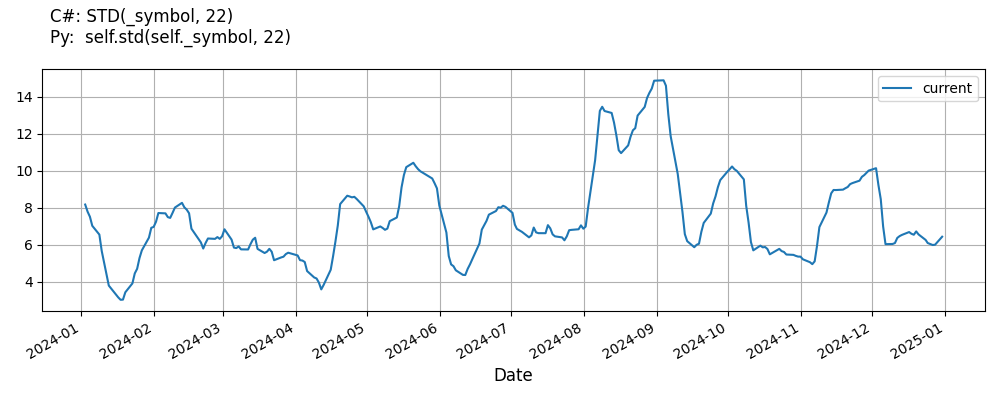# Supported Indicators

## Standard Deviation

### Introduction

This indicator computes the n-period population standard deviation.

To view the implementation of this indicator, see the LEAN GitHub repository.

### Using STD Indicator

To create an automatic indicators for StandardDeviation, call the STD helper method from the QCAlgorithm class. The STD method creates a StandardDeviation object, hooks it up for automatic updates, and returns it so you can used it in your algorithm. In most cases, you should call the helper method in the Initialize method.

public class StandardDeviationAlgorithm : QCAlgorithm
{
private Symbol _symbol;
private StandardDeviation _std;

public override void Initialize()
{
_std = STD(_symbol, 22);
}

public override void OnData(Slice data)
{
{
// The current value of _std is represented by itself (_std)
// or _std.Current.Value
Plot("StandardDeviation", "std", _std);

}
}
}
class StandardDeviationAlgorithm(QCAlgorithm):
def Initialize(self) -> None:
self.std = self.STD(self.symbol, 22)

def OnData(self, slice: Slice) -> None:
# The current value of self.std is represented by self.std.Current.Value
self.Plot("StandardDeviation", "std", self.std.Current.Value)



The following reference table describes the STD method:

### STD()1/1

            StandardDeviation QuantConnect.Algorithm.QCAlgorithm.STD (
Symbol                           symbol,
Int32                            period,
*Nullable<Resolution>      resolution,
*Func<IBaseData, Decimal>  selector
)


Creates a new StandardDeviation indicator. This will return the population standard deviation of samples over the specified period.

If you don't provide a resolution, it defaults to the security resolution. If you provide a resolution, it must be greater than or equal to the resolution of the security. For instance, if you subscribe to hourly data for a security, you should update its indicator with data that spans 1 hour or longer.

You can manually create a StandardDeviation indicator, so it doesn't automatically update. Manual indicators let you update their values with any data you choose.

Updating your indicator manually enables you to control when the indicator is updated and what data you use to update it. To manually update the indicator, call the Update method with time/number pair, or an IndicatorDataPoint. The indicator will only be ready after you prime it with enough data.

public class StandardDeviationAlgorithm : QCAlgorithm
{
private Symbol _symbol;
private StandardDeviation _std;

public override void Initialize()
{
_std = new StandardDeviation(22);
}

public override void OnData(Slice data)
{
if (data.Bars.TryGeValue(_symbol, out var bar))
{
_std.Update(bar.EndTime, bar.Close);
}

{
// The current value of _std is represented by itself (_std)
// or _std.Current.Value
Plot("StandardDeviation", "std", _std);

}
}
}
class StandardDeviationAlgorithm(QCAlgorithm):
def Initialize(self) -> None:
self.std = StandardDeviation(22)

def OnData(self, slice: Slice) -> None:
bar = slice.Bars.get(self.symbol)
if bar:
self.std.Update(bar.EndTime, bar.Close)

# The current value of self.std is represented by self.std.Current.Value
self.Plot("StandardDeviation", "std", self.std.Current.Value)



To register a manual indicator for automatic updates with the security data, call the RegisterIndicator method.

public class StandardDeviationAlgorithm : QCAlgorithm
{
private Symbol _symbol;
private StandardDeviation _std;

public override void Initialize()
{
_std = new StandardDeviation(22);
RegisterIndicator(_symbol, _std, Resolution.Daily);
}

public override void OnData(Slice data)
{
{
// The current value of _std is represented by itself (_std)
// or _std.Current.Value
Plot("StandardDeviation", "std", _std);

}
}
}
class StandardDeviationAlgorithm(QCAlgorithm):
def Initialize(self) -> None:
self.std = StandardDeviation(22)
self.RegisterIndicator(self.symbol, self.std, Resolution.Daily)

def OnData(self, slice: Slice) -> None:
# The current value of self.std is represented by self.std.Current.Value
self.Plot("StandardDeviation", "std", self.std.Current.Value)



The following reference table describes the StandardDeviation constructor:

### StandardDeviation()1/2

            StandardDeviation QuantConnect.Indicators.StandardDeviation (
int  period
)


On a data set of size N will use an N normalizer and would thus be biased if applied to a subset.

### StandardDeviation()2/2

            StandardDeviation QuantConnect.Indicators.StandardDeviation (
string  name,
int     period
)


On a data set of size N will use an N normalizer and would thus be biased if applied to a subset.

### Visualization

The following image shows plot values of selected properties of StandardDeviation using the plotly library.You can also see our Videos. You can also get in touch with us via Discord.